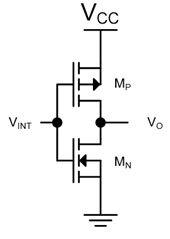If you have a related question, please click the "Ask a related question" button in the top right corner. The newly created question will be automatically linked to this question.

# SN74LVC1G08: Impedance on Y

Part Number: SN74LVC1G08

Hi,
im trying to match impedance on my circuit and wanted to know how much impedance there is on output Y (SN74LVC1G08DBV)...didn´t find something in the Datasheet...so is it near 0 ohm?

• You can derive the worst-case output impedance from VOL and IOL.
The typical output impedance is roughly half that.

• Thank you for the answer. So to fully understand it: In my case V_OL is 0.55V and I_OL = 32 mA. Than I get a impedance of roughly 17 ohm --> half of that is 8.5 ohm. Is that what you meant?
Why is the output impedance half?

• Hi Viktor,

It just happens to work out that way. The datasheet value of 0.55V provided is a maximum limit, which means that you should _never_ see this device actually output 0.55V, including variations from the manufacturing process and across the full temperature range. We usually guard band these specs a bit on top of them being measured across all possible variations of the device to avoid any possible liability for any outlier devices.

Most devices you will find have a typical output resistance about half of what is shown in the datasheet for the 'worst case' value. If you're designing a system, it's best to design to the worst case value, but if you want to know "about how a device performs" then the typical value is more useful.

• Hi Emrys,

and what about the case when the output is high....so in the datasheet V_OH = min. 3.8V (32mA)...than I have roughtly 118 ohm. Is it here also half of the calculated value (59 ohm)? In conclusion, I will get a impedance of 8.5 ohm at low level and 59 ohm impedance on high level?